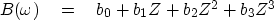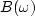Next: SYMMETRIES Up: INVERTIBLE SLOW FT PROGRAM Previous: Truncation problems

## FT by Z-transform

The program slowft() is unnecessarily slow, requiring us to compute a complex exponential at each step. By reorganizing easily using the Z-transform, the computational load can be reduced by about a factor of five (from a complex exponential to a complex multiply) at every step.

For simplicity we consider a signal that is only four points long:(10)
Reorganizing the polynomial (10) by nesting gives(11)
A subroutine for evaluatingin this way is polyft().

# Fourier transform by polynomial evaluation.
subroutine polyft( nt,tt, nw,cww )
integer nt      # number of points in the time domain
integer nw      # number of points in the fourier transform
real    tt(nt)  # sampled function of time
complex cww(nw) # sampled fourier transform
integer it, iw
real omega
complex cz, cw
do iw= 1, nw {
omega =  3.14159265 * (iw-1.) / ( nw-1.)
cz = cexp( cmplx( 0., omega ) )
cw = tt(nt)
do it= nt-1, 1, -1      # loop runs backwards
cw = cw * cz + tt(it)
cww(iw) = cw
}
return; endNext: SYMMETRIES Up: INVERTIBLE SLOW FT PROGRAM Previous: Truncation problems
Stanford Exploration Project
10/21/1998